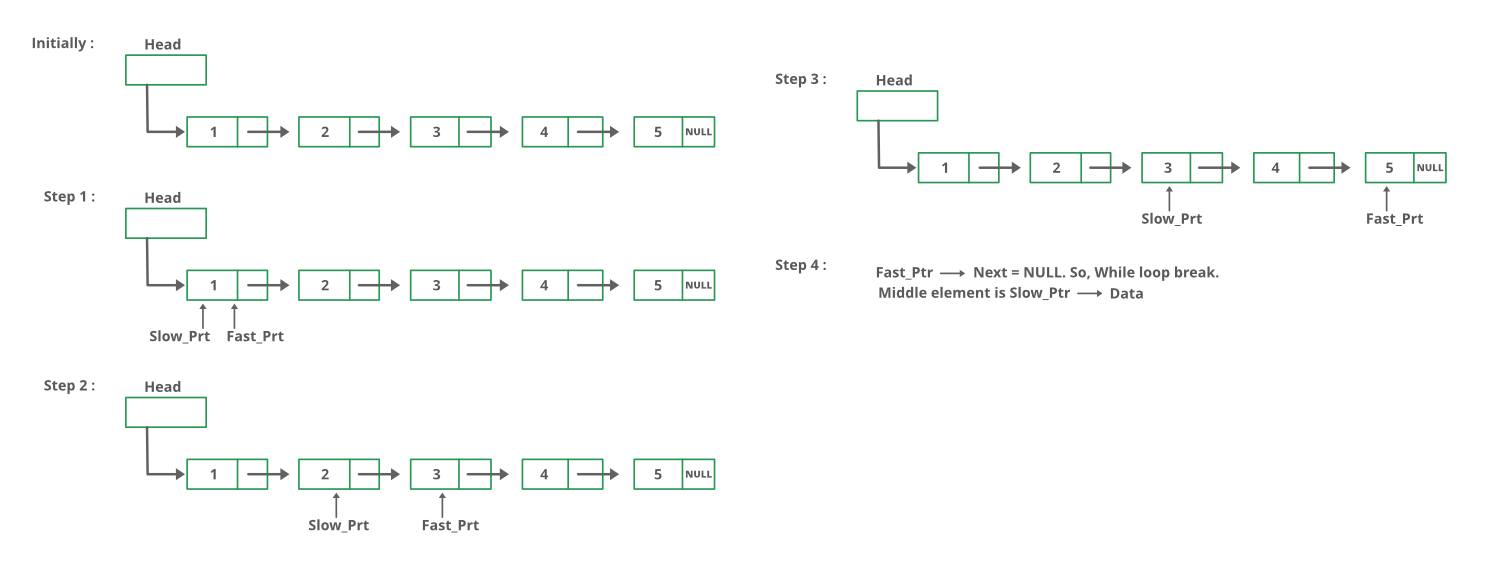GeeksforGeeks App
Open AppBrowser
Continue

# Java Program For Finding The Middle Element Of A Given Linked List

Given a singly linked list, find the middle of the linked list. For example, if the given linked list is 1->2->3->4->5 then the output should be 3.
If there are even nodes, then there would be two middle nodes, we need to print the second middle element. For example, if given linked list is 1->2->3->4->5->6 then the output should be 4.

Method 1:
Traverse the whole linked list and count the no. of nodes. Now traverse the list again till count/2 and return the node at count/2.

## Java

 `// Java Program for the above approach``import` `java.io.*;``class` `GFG {`` ` `    ``Node head;`` ` `    ``// Creating a new Node``    ``class` `Node {``        ``int` `data;``        ``Node next;``        ``public` `Node(``int` `data)``        ``{``            ``this``.data = data;``            ``this``.next = ``null``;``        ``}``    ``}`` ` `    ``// Function to add a new Node``    ``public` `void` `pushNode(``int` `data)``    ``{``        ``Node new_node = ``new` `Node(data);``        ``new_node.next = head;``        ``head = new_node;``    ``}`` ` `    ``// Displaying the elements in the list``    ``public` `void` `printNode()``    ``{``        ``Node temp = head;``        ``while` `(temp != ``null``) {``            ``System.out.print(temp.data + ``"->"``);``            ``temp = temp.next;``        ``}``        ``System.out.print(``"Null"` `+ ``"\n"``);``    ``}`` ` `    ``// Finding the length of the list.``    ``public` `int` `getLen()``    ``{``        ``int` `length = ``0``;``        ``Node temp = head;``        ``while` `(temp != ``null``) {``            ``length++;``            ``temp = temp.next;``        ``}``        ``return` `length;``    ``}`` ` `    ``// Printing the middle element of the list.``    ``public` `void` `printMiddle()``    ``{``        ``if` `(head != ``null``) {``            ``int` `length = getLen();``            ``Node temp = head;``            ``int` `middleLength = length / ``2``;``            ``while` `(middleLength != ``0``) {``                ``temp = temp.next;``                ``middleLength--;``            ``}``            ``System.out.print(``"The middle element is ["` `+ temp.data + ``"]"``);``            ``System.out.print(``"\n\n"``);``        ``}``    ``}`` ` `    ``public` `static` `void` `main(String[] args)``    ``{``        ``GFG list = ``new` `GFG();``        ``for` `(``int` `i = ``5``; i >= ``1``; i--) {``            ``list.pushNode(i);``            ``list.printNode();``            ``list.printMiddle();``        ``}``    ``}``}``// This Code is contributed by Yash Agarwal`

Time Complexity: O(n) where n is no of nodes in linked list
Auxiliary Space: O(1)

Method 2:
Traverse linked list using two pointers. Move one pointer by one and the other pointers by two. When the fast pointer reaches the end slow pointer will reach the middle of the linked list.

Below image shows how printMiddle function works in the code :## Java

 `// Java program to find middle of``// the linked list``class` `LinkedList``{``    ``// Head of linked list``    ``Node head;` `    ``// Linked list node``    ``class` `Node``    ``{``        ``int` `data;``        ``Node next;``        ``Node(``int` `d)``        ``{``            ``data = d;``            ``next = ``null``;``        ``}``    ``}` `    ``// Function to print middle of``    ``// the linked list``    ``void` `printMiddle()``    ``{``        ``Node slow_ptr = head;``        ``Node fast_ptr = head;``        ``if` `(head != ``null``)``        ``{``            ``while` `(fast_ptr != ``null` `&&``                   ``fast_ptr.next != ``null``)``            ``{``                ``fast_ptr = fast_ptr.next.next;``                ``slow_ptr = slow_ptr.next;``            ``}``            ``System.out.println(``"The middle element is ["` `+``                                ``slow_ptr.data + ``"]"``);``        ``}``    ``}` `    ``// Inserts a new Node at front of the list.``    ``public` `void` `push(``int` `new_data)``    ``{``        ``/* 1 & 2: Allocate the Node &``                  ``Put in the data*/``        ``Node new_node = ``new` `Node(new_data);` `        ``// 3. Make next of new Node as head``        ``new_node.next = head;` `        ``// 4. Move the head to point to new Node``        ``head = new_node;``    ``}` `    ``// This function prints contents of linked list``    ``// starting from  the given node``    ``public` `void` `printList()``    ``{``        ``Node tnode = head;``        ``while` `(tnode != ``null``)``        ``{``            ``System.out.print(tnode.data + ``"->"``);``            ``tnode = tnode.next;``        ``}``        ``System.out.println(``"NULL"``);``    ``}`` ` `    ``// Driver code``    ``public` `static` `void` `main(String [] args)``    ``{``        ``LinkedList llist = ``new` `LinkedList();``        ``for` `(``int` `i = ``5``; i > ``0``; --i)``        ``{``            ``llist.push(i);``            ``llist.printList();``            ``llist.printMiddle();``        ``}``    ``}``}``// This code is contributed by Rajat Mishra`

Output:

```5->NULL
The middle element is 

4->5->NULL
The middle element is 

3->4->5->NULL
The middle element is 

2->3->4->5->NULL
The middle element is 

1->2->3->4->5->NULL
The middle element is ```

Time Complexity: O(n) where n is the number of nodes in the given linked list.
Auxiliary Space: O(1), no extra space is required, so it is a constant.

Method 3:
Initialize mid element as head and initialize a counter as 0. Traverse the list from head, while traversing increment the counter and change mid to mid->next whenever the counter is odd. So the mid will move only half of the total length of the list.
Thanks to Narendra Kangralkar for suggesting this method.

## Java

 `// Java program to implement the``// above approach``class` `GFG``{``    ``static` `Node head;` `    ``// Link list node``    ``class` `Node``    ``{``        ``int` `data;``        ``Node next;` `        ``// Constructor``        ``public` `Node(Node next,``                    ``int` `data)``        ``{``            ``this``.data = data;``            ``this``.next = next;``        ``}``    ``}` `    ``// Function to get the middle of``    ``// the linked list``    ``void` `printMiddle(Node head)``    ``{``        ``int` `count = ``0``;``        ``Node mid = head;` `        ``while` `(head != ``null``)``        ``{``            ``// Update mid, when 'count'``            ``// is odd number``            ``if` `((count % ``2``) == ``1``)``                ``mid = mid.next;` `            ``++count;``            ``head = head.next;``        ``}` `        ``// If empty list is provided``        ``if` `(mid != ``null``)``            ``System.out.println(``"The middle element is ["` `+``                                ``mid.data + ``"]\n"``);``    ``}` `    ``void` `push(Node head_ref, ``int` `new_data)``    ``{``    ` `        ``// Allocate node``        ``Node new_node = ``new` `Node(head_ref,``                                 ``new_data);` `        ``// Move the head to point to the new node``        ``head = new_node;``    ``}` `    ``// A utility function to print a``    ``// given linked list``    ``void` `printList(Node head)``    ``{``        ``while` `(head != ``null``)``        ``{``            ``System.out.print(head.data + ``"-> "``);``            ``head = head.next;``        ``}``        ``System.out.println(``"null"``);``    ``}` `    ``// Driver code``    ``public` `static` `void` `main(String[] args)``    ``{``        ``GFG ll = ``new` `GFG();` `        ``for``(``int` `i = ``5``; i > ``0``; i--)``        ``{``            ``ll.push(head, i);``            ``ll.printList(head);``            ``ll.printMiddle(head);``        ``}``    ``}``}``// This code is contributed by mark_3`

Output:

```5->NULL
The middle element is 

4->5->NULL
The middle element is 

3->4->5->NULL
The middle element is 

2->3->4->5->NULL
The middle element is 

1->2->3->4->5->NULL
The middle element is ```

Time Complexity: O(n) where n is the number of nodes in the given linked list.
Auxiliary Space: O(1), no extra space is required, so it is a constant.

Please refer complete article on Find the middle of a given linked list for more details!

My Personal Notes arrow_drop_up